# 一：运行截图

## 1.二进制转其他进制，及错误示范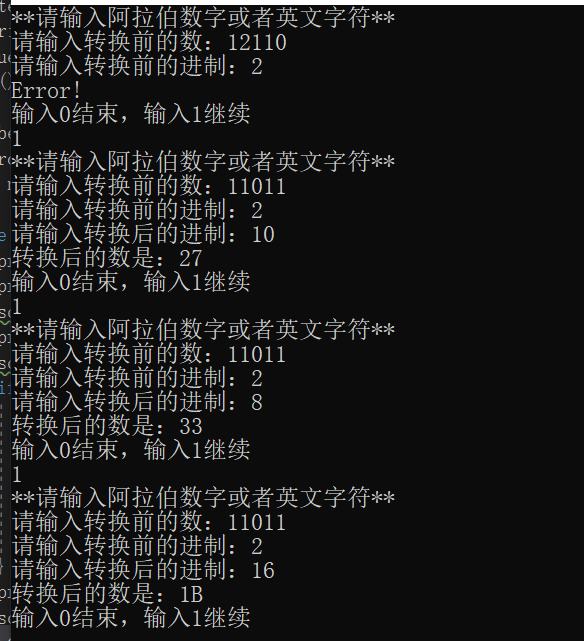## 2.八进制转其他进制，及错误示范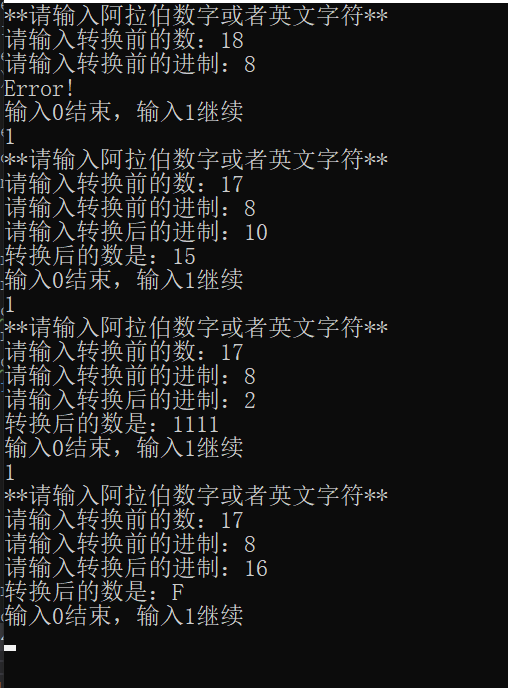## 3.十进制转其他进制，及错误示范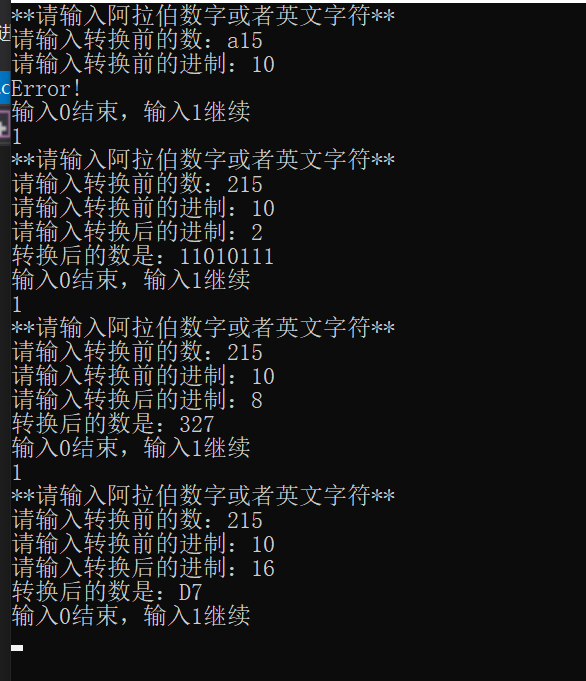## 4.十六进制转其他进制，及错误示范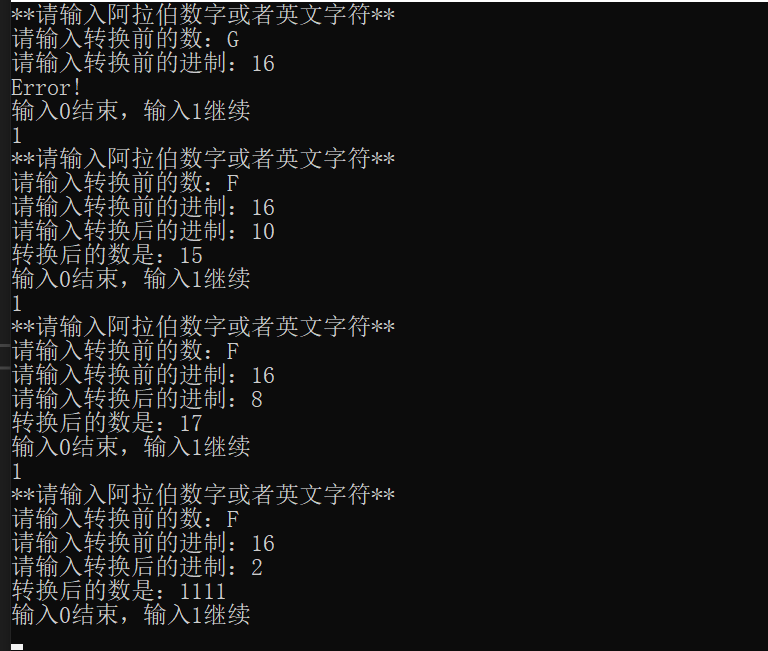# 二：所有函数及其功能

#### 1.char num_to_char(int n)函数

char num_to_char(int n)
{
if (n >= 0 && n <= 9){
return (char)('0' + n - 0);
}
else{
return (char)('A' + n - 10);
}
}//该函数用于将数字转成相应的字符


#### 2.int char_to_num(char a)函数

int char_to_num(char a)
{
if (a >= '0' && a <= '9'){
return a - '0';
}
else{
return a - 'A' + 10;
}
}//该函数用于将字符转成相应的数字


#### 3.int decimal(char n[], int before)函数

int decimal(char n[], int before)
{
int Decimal = 0;
int len，i;
for (i = 0; n[i] != '\0'; i++);
len = i;
for (i = 0; i <= len - 1; i++){
Decimal = (Decimal * before) + char_to_num(n[i]);
}
return Decimal;
}//该函数用于将其他进制数统一转换成十进制数


#### 4.int Toafter(char n[], int Decimal, int after)函数

int Toafter(char n[], int Decimal, int after)
{
int i = 0;
while (Decimal)
{
n[i] = num_to_char(Decimal % after);
Decimal /= after;
i++;
}
n[i] = '\0';
return i;
}//该函数用于将转化后的十进制数转化换成所需要的进制数



#### 5.void reprint(char n[], int len)函数

void reprint(char n[], int len)
{
int i;
for (i = len - 1; i >= 0; i--){
printf("%c", n[i]);
}
printf("\n");
}//该函数用于逆序输出所需要的进制数


#### 6.int istrue(char x[], int before) 函数

/*该函数用于判断输入的进制数是否正确*/
int istrue(char x[], int before) {
int flag = 1;
int length = strlen(x);
int i = 0;
if (before == 2) {
do {
if (x[i] != 49 && x[i] != 48) {
printf("Error!\n");
flag = 0;
break;
}
i++;
} while (i < length);
}//判断输入对二进制数是否正确
else if (before == 8) {
do {
if (!(x[i] >= 48 && x[i] <= 55)) {
printf("Error!\n");
flag = 0;
break;
}
i++;
} while (i < length);
}//判断输入的八进制数是否正确
else if (before == 10) {
do {
if (!(x[i] >= 48 && x[i] <= 57)) {
printf("Error!\n");
flag = 0;
break;
}
i++;
} while (i < length);
}//判断输入的十进制数是否正确
else {
do {
if (!(x[i] >= 48 && x[i] <= 57) && !(x[i] >= 65 && x[i] <= 70) && !(x[i] >= 97 && x[i] <= 102)) {
printf("Error!\n");
flag = 0;
break;
}
i++;
} while (i < length);
}//判断输入的十六进制数是否正确
return flag;
}


# 三：宏定义

#define MAX 200


# 四：展示main函数

int main()
{
int before, after, length, Decimal, flag;
int roll = 1;
char n[MAX], x[MAX];

while (roll == 1) {
printf("**请输入阿拉伯数字或者英文字符**\n");
printf("请输入转换前的数：");
scanf("%s", &n);
printf("请输入转换前的进制：");
scanf("%d", &before);
//if语句为判断输入是否正确，如果正确则进行转换
if (istrue(n, before) == 1) {
printf("请输入转换后的进制：");
scanf("%d", &after);
printf("转换后的数是：");
Decimal = decimal(n, before);
length = Toafter(n, Decimal, after);
reprint(n, length);
}
printf("输入0结束，输入1继续\n");
scanf("%d", &roll);
}
return 0;
}


# 五：思维导图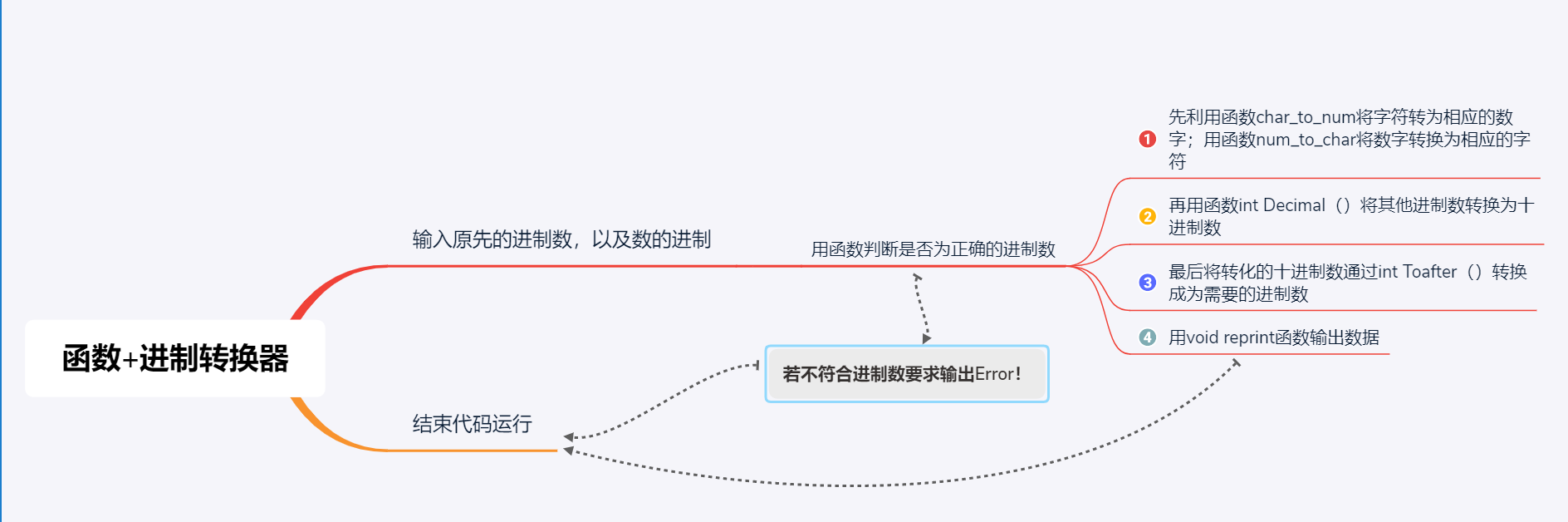# 六：遇到的问题及解决方案

#### 1.问题：

int istrue()函数刚开始写的时候使整个程序都乱了

# 七：代码互评

int main()
{
printf("\n****欢迎使用进制转换器****\n");
//...此处为我删减的她的变量定义部分
while (flag) {
begin:printf("\n请输入您需要转换的数：");
scanf("%s", op);
printf("\n该数的原进制为： ");
scanf("%d", &origin);
if (inspect(op, origin) == 0)
{
error:printf("\n您输入的数据有误\n\n重新输入请按 1 ，结束请按 0 \n");
int sign;
scanf("%d", &sign);
if (sign)
{
goto begin;
}
else
{
goto last;
}
}
else if (inspect(op, origin))
{

printf("\n转换的目标进制为： ");
scanf("%d", &objective);
if (origin == 16)
{
}
else
{
integer = wantonly_to_decimalism(atoi(op), origin);
}
switch (objective)
{
case 2:
result = decimalism_to_binary(integer);
printf("\n该数的%d进制数为:%d", objective, result);
break;
//...此处为我的删减部分
default:goto error; break;
}
}
printf("\n继续请按 1 ，退出按 0 .\n");
scanf("%d", &flag);
if (flag == 0) { goto last; }
system("cls");
}
last:printf("****谢谢使用本进制转换器****\n");
return 0;
}


# 八：总结

#### 函数概念的理解：

posted @ 2019-11-10 20:34  羊腿桂鱼yu  阅读(197)  评论(0编辑  收藏  举报